## Which important property did Mendeleev use to classify the elements in his periodic table and did he stick to that?

Mendeleev arranged the elements in his periodic table ordered by atomic weight or mass. He arranged the elements in periods and groups in order of their increasing atomic weight. He placed the elements with similar properties in the same group.
However, he did not stick to this arrangement for long. He found out that if the elements were arranged strictly in order of their increasing atomic weights, then some elements did not fit within this scheme of classification.
Therefore, he ignored the order of atomic weights in some cases. For example, the atomic weight of iodine is lower than that of tellurium. Still Mendeleev placed tellurium (in Group VI) before iodine (in Group VII) simply because iodine’s properties are so similar tofluorine, chlorine, and bromine.

## What is the basic theme of organisation in the periodic table?

The basic theme of organisation of elements in the periodic table is to classify the elements in periods and groups according to their properties. This arrangement makes the study of elements and their compounds simple and systematic. In the periodic table, elements with similar properties are placed in the same group.

## (iv) {1, 3, 5, 7, 9}

(i) All the elements of this set are natural numbers as well as the divisors of 6.
Therefore, (i) matches with (c).
(ii) It can be seen that 2 and 3 are prime numbers. They are also the divisors of 6. Therefore, (ii) matches with (a).
(iii) All the elements of this set are letters of the word MATHEMATICS. Therefore, (iii) matches with (d).
(iv) All the elements of this set are odd natural numbers less than 10. Therefore, (iv) matches with (b).

## (vi) F = {x: x is a consonant in the English alphabet which proceeds k}.

(i) A = {x: x is an odd natural number} = {1, 3, 5, 7, 9 …}
(ii) B = {x: x is an integer;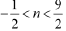}
It can be se
(ii) B = {x: x is an integer; }
It can be seen that -1/2 = - 0.5 and 9/2 = 5
∴ B = {0,1,2,3,4}
(iii) C = {x: x is an integer;x 2≤4}
It can be seen that
(-1)2 = 1 ≤ 4; (-2)2 = 4 ≤ 4; (-3)2 = 9 > 4
02 = 0 ≤ 4
12 = 1 ≤ 4
22 = 4 ≤ 4
32 = 9 > 4
∴C = {-2, -1, 0, 1, 2}
(iv) D = (x: x is a letter in the word “LOYAL”) = {L, O, Y, A}
(v) E = {x: x is a month of a year not having 31 days}
= {February, April, June, September, November}
(vi) F = {x: x is a consonant in the English alphabet which precedes k}
= {b, c, d, f, g, h, j}

## (v) {1, 4, 9 … 100}

(i) {3, 6, 9, 12} = {x: x = 3n, n∈ N and 1 ≤ n ≤ 4} (ii) {2, 4, 8, 16, 32}
It can be seen that 2 = 21, 4 = 22, 8 = 23, 16 = 24, and 32 = 25. ∴ {2, 4, 8, 16, 32} = {x: x = 2 n, n∈ N and 1 ≤ n ≤ 5}
(iii) {5, 25, 125, 625}
It can be seen that 5 = 51, 25 = 52, 125 = 53, and 625 = 54. ∴ {5, 25, 125, 625} = {x: x = 5n, n∈N and 1 ≤ n ≤ 4}
(iv) {2, 4, 6 …}
It is a set of all even natural numbers.
∴ {2, 4, 6 …} = {x: x is an even natural number}
(v) {1, 4, 9 … 100}
It can be seen that 1 = 12, 4 = 22, 9 = 32 …100 = 102.
∴ {1, 4, 9… 100} = {x: x = n2, n∈N and 1 ≤ n ≤ 10}

## Write the following sets in roster form:(i) A = {x: x is an integer and –3 < x < 7}.(ii) B = {x: x is a natural number less than 6}.(iii) C = {x: x is a two-digit natural number such that the sum of its digits is 8}(iv) D = {x: x is a prime number which is divisor of 60}.(v) E = The set of all letters in the word TRIGONOMETRY.(vi) F = The set of all letters in the word BETTER.

(i) A = {x: x is an integer and –3 < x < 7}
The elements of this set are –2, –1, 0, 1, 2, 3, 4, 5, and 6 only.
Therefore, the given set can be written in roster form as
A = {–2, –1, 0, 1, 2, 3, 4, 5, 6}
(ii) B = {x: x is a natural number less than 6}
The elements of this set are 1, 2, 3, 4, and 5 only.
Therefore, the given set can be written in roster form as
B = {1, 2, 3, 4, 5}
(iii) C = {x: x is a two-digit natural number such that the sum of its digits is 8}
The elements of this set are 17, 26, 35, 44, 53, 62, 71, and 80 only.
Therefore, this set can be written in roster form as
C = {17, 26, 35, 44, 53, 62, 71, 80}
(iv) D = {x: x is a prime number which is a divisor of 60}
∴60 = 2 × 2 × 3 × 5
The elements of this set are 2, 3, and 5 only.
Therefore, this set can be written in roster form as D = {2, 3, 5}.
(v) E = The set of all letters in the word TRIGONOMETRY
There are 12 letters in the word TRIGONOMETRY, out of which letters T, R, and O are
repeated.
Therefore, this set can be written in roster form as
E = {T, R, I, G, O, N, M, E, Y}
(vi) F = The set of all letters in the word BETTER
There are 6 letters in the word BETTER, out of which letters E and T are repeated.
Therefore, this set can be written in roster form as

### Let A = {1, 2, 3, 4, 5, 6}. Insert the appropriate symbol ∈or ∉ in the blank spaces:

Let A = {1, 2, 3, 4, 5, 6}. Insert the appropriate symbol ∈or ∉ in the blank spaces:
(i) 5…A (ii) 8…A (iii) 0…A
(iv) 4…A (v) 2…A (vi) 10…A

(i) 5 ∈ A
(ii) 8 ∉ A
(iii) 0 ∉ A
(iv) 4 ∈ A
(v) 2 ∈ A
(vi) 10 ∉ A

### Which of the following are sets? Justify our answer.

Which of the following are sets? Justify our answer.
(i) The collection of all months of a year beginning with the letter J.
(ii) The collection of ten most talented writers of India.
(iii) A team of eleven best-cricket batsmen of the world.
(iv) The collection of all boys in your class.
(v) The collection of all natural numbers less than 100.
(vi) A collection of novels written by the writer Munshi Prem Chand.
(vii) The collection of all even integers.
(viii) The collection of questions in this Chapter.
(ix) A collection of most dangerous animals of the world.

(i) The collection of all months of a year beginning with the letter J is a well-defined
collection of objects because one can definitely identify a month that belongs to this
collection.
Hence, this collection is a set.
(ii) The collection of ten most talented writers of India is not a well-defined collection
because the criteria for determining a writer’s talent may vary from person to person.
Hence, this collection is not a set.
(iii) A team of eleven best cricket batsmen of the world is not a well-defined collection
because the criteria for determining a batsman’s talent may vary from person to person.
Hence, this collection is not a set.
(iv) The collection of all boys in your class is a well-defined collection because you can
definitely identify a boy who belongs to this collection.
Hence, this collection is a set.
(v) The collection of all natural numbers less than 100 is a well-defined collection
because one can definitely identify a number that belongs to this collection.
Hence, this collection is a set.
(vi) A collection of novels written by the writer Munshi Prem Chand is a well-defined
collection because one can definitely identify a book that belongs to this collection.
Hence, this collection is a set.
(vii) The collection of all even integers is a well-defined collection because one can
definitely identify an even integer that belongs to this collection.
Hence, this collection is a set.
(viii) The collection of questions in this chapter is a well-defined collection because one
can definitely identify a question that belongs to this chapter.
Hence, this collection is a set.
(ix) The collection of most dangerous animals of the world is not a well-defined
collection because the criteria for determining the dangerousness of an animal can vary
from person to person.
Hence, this collection is not a set.

## Chapter -4 Mathematical Induction

### The Principle of Mathematical Induction

Suppose there is a given statement P(n) involving the natural number n such that
(i) The statement is true for n = 1, i.e., P(1) is true, and
(ii) If the statement is true for n = k (where k is some positive integer), then the statement is also true for n = k + 1, i.e., truth of P(k) implies the truth of P (k + 1).
Then, P(n) is true for all natural numbers n.

## Exercise solutions

15. ### 12 + 32 + 52 + …+ (2n-1)2 = n (2 n − 1) (2 n + 1)/3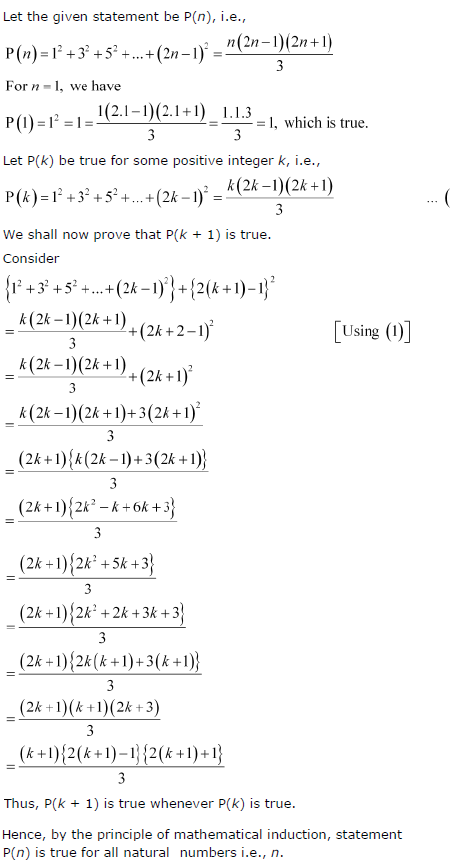## Wednesday, 3 July 2013

### How many sub-shells are associated with n = 4

(a) How many sub-shells are associated with n = 4?
(b) How many electrons will be present in the sub-shells having ms value of -1/2 for n = 4?
(a) n = 4 (Given)
For a given value of ‘n’, ‘l’ can have values from zero to (n - 1). ∴ l = 0, 1, 2, 3
Thus, four sub-shells are associated with n = 4, which are s, p, d and f.
(b) Number of orbitals in the nth shell = n2
For n = 4
Number of orbitals = 16
If each orbital is taken fully, then it will have 1 electron with ms value of-1/2
∴ Number of electrons with ms value of -1/2 is 16.

### Indicate the number of unpaired electrons in: (a) P, (b) Si, (c) Cr, (d) Fe and (e) Kr.

Question 2.66: Indicate the number of unpaired electrons in: (a) P, (b) Si, (c) Cr, (d) Fe and (e) Kr.
(a) Phosphorus (P):   Atomic number = 15
The electronic configuration of P is:
1s2 2s2 2p6 3s2 3p3
The orbital picture of P can be represented as: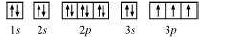From the orbital picture, phosphorus has three unpaired electrons.
(b) Silicon (Si):
Atomic number = 14
The electronic configuration of Si is:
1s2 2s2 2p6 3s2 3p2
The orbital picture of Si can be represented as: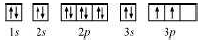From the orbital picture, silicon has two unpaired electrons.
(c) Chromium (Cr):
Atomic number = 24
The electronic configuration of Cr is:
1s2 2s2 2p6 3s2 3p6 4s1 3d5
The orbital picture of chromium is: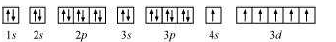From the orbital picture, chromium has six unpaired electrons.
(d) Iron (Fe):
Atomic number = 26
The electronic configuration is:
1s2 2s2 2p6 3s2 3p6 4s2 3d6
The orbital picture of chromium is: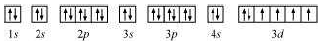From the orbital picture, iron has four unpaired electrons.
(e) Krypton (Kr):
Atomic number = 36
The electronic configuration is:
1s2 2s2 2p6 3s2 3p6 4s2 3d10 4p 6
The orbital picture of krypton is: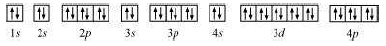Since all orbitals are fully occupied, there are no unpaired electrons in krypton.